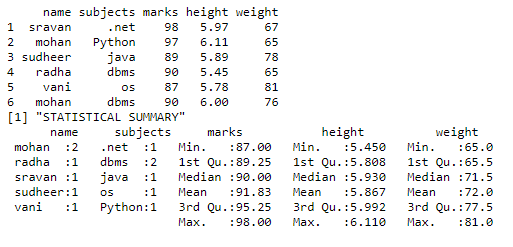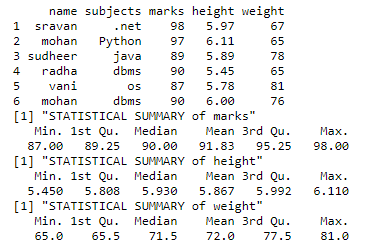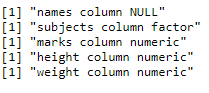Open in App
Not now

# Get the statistical summary and nature of the DataFrame in R

• Last Updated : 07 Apr, 2021

In this article, we will see how to find the statistics of the given data frame. We will use the summary() function to get the statistics for each column:

Syntax: summary(dataframe_name)

The result produced will contain the following details:

• Minimum value – returns the minimum value from each column
• Maximum value – returns the maximum value from each column
• Mean – returns the mean value from each column
• Median – returns the median from each column
• 1st quartile – returns the 1st quartile from each column
• 3rd quartile – returns the 3rd quartile from each column.

Example 1: In this example data, we had taken student marks, height, weight, and marks, so we are calculating the summary of that two columns.

## R

 `# create vector with names``name = ``c``(``"sravan"``, ``"mohan"``, ``"sudheer"``, ``         ``"radha"``, ``"vani"``, ``"mohan"``)`` ` `# create vector with subjects``subjects = ``c``(``".net"``, ``"Python"``, ``"java"``,``             ``"dbms"``, ``"os"``, ``"dbms"``)`` ` `# create a vector with marks``marks = ``c``(98, 97, 89, 90, 87, 90)`` ` `# create vector with height``height = ``c``(5.97, 6.11, 5.89, 5.45, 5.78, 6.0)`` ` `# create vector with weight``weight = ``c``(67, 65, 78, 65, 81, 76)`` ` `# pass these vectors to the data frame``data = ``data.frame``(name, subjects,``                  ``marks, height, weight)`` ` `# display``print``(data)``print``(``"STATISTICAL SUMMARY"``)`` ` `# use summary function ``print``(``summary``(data))`

Output:Example 2: In this example, we are getting a statistical summary of individual columns

## R

 `# create vector with names``name = ``c``(``"sravan"``,``"mohan"``,``"sudheer"``,``         ``"radha"``,``"vani"``,``"mohan"``)`` ` `# create vector with subjects``subjects = ``c``(``".net"``,``"Python"``,``"java"``,``             ``"dbms"``,``"os"``,``"dbms"``)`` ` `# create a vector with marks``marks=``c``(98,97,89,90,87,90)`` ` `# create vector with height``height=``c``(5.97,6.11,5.89,``         ``5.45,5.78,6.0)`` ` `# create vector with weight``weight=``c``(67,65,78,65,81,76)`` ` `# pass these vectors to the data frame``data=``data.frame``(name,subjects,marks,``                ``height,weight)`` ` `# display``print``(data)``print``(``"STATISTICAL SUMMARY of marks"``)`` ` `# use summary function  on marks column``print``(``summary``(data\$marks))``print``(``"STATISTICAL SUMMARY of height"``)`` ` ` ` `# use summary function on height column``print``(``summary``(data\$height))``print``(``"STATISTICAL SUMMARY of weight"``)`` ` `# use summary function on weight column``print``(``summary``(data\$weight))`

Output:### Finding Nature of the data frame:

We can use class() function to get the nature of the dataframe.

It will return:

• Either data is NULL or not
• The datatype of a particular column in a dataframe

Syntax: class(dataframe\$column_name)

Example:

## R

 `# create vector with names``name = ``c``(``"sravan"``,``"mohan"``,``"sudheer"``,``         ``"radha"``,``"vani"``,``"mohan"``)`` ` `# create vector with subjects``subjects = ``c``(``".net"``,``"Python"``,``"java"``,``             ``"dbms"``,``"os"``,``"dbms"``)`` ` `# create a vector with marks``marks=``c``(98,97,89,90,87,90)`` ` `# create vector with height``height=``c``(5.97,6.11,5.89,``         ``5.45,5.78,6.0)`` ` `# create vector with weight``weight=``c``(67,65,78,65,81,76)`` ` `# pass these vectors to the data frame``data=``data.frame``(name,subjects,marks,``                ``height,weight)`` ` `# nature of dataframe``print``(``paste``(``"names column"``,``class``(data\$names)))``print``(``paste``(``"subjects column"``,``class``(data\$subjects)))``print``(``paste``(``"marks column"``,``class``(data\$marks)))``print``(``paste``(``"height column"``,``class``(data\$height)))``print``(``paste``(``"weight column"``,``class``(data\$weight)))`

Output:My Personal Notes arrow_drop_up